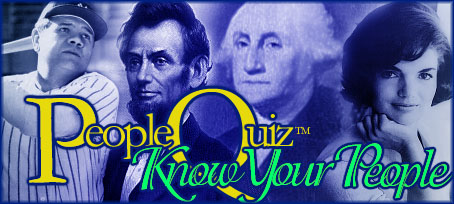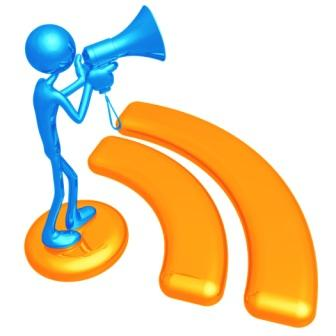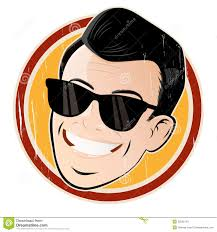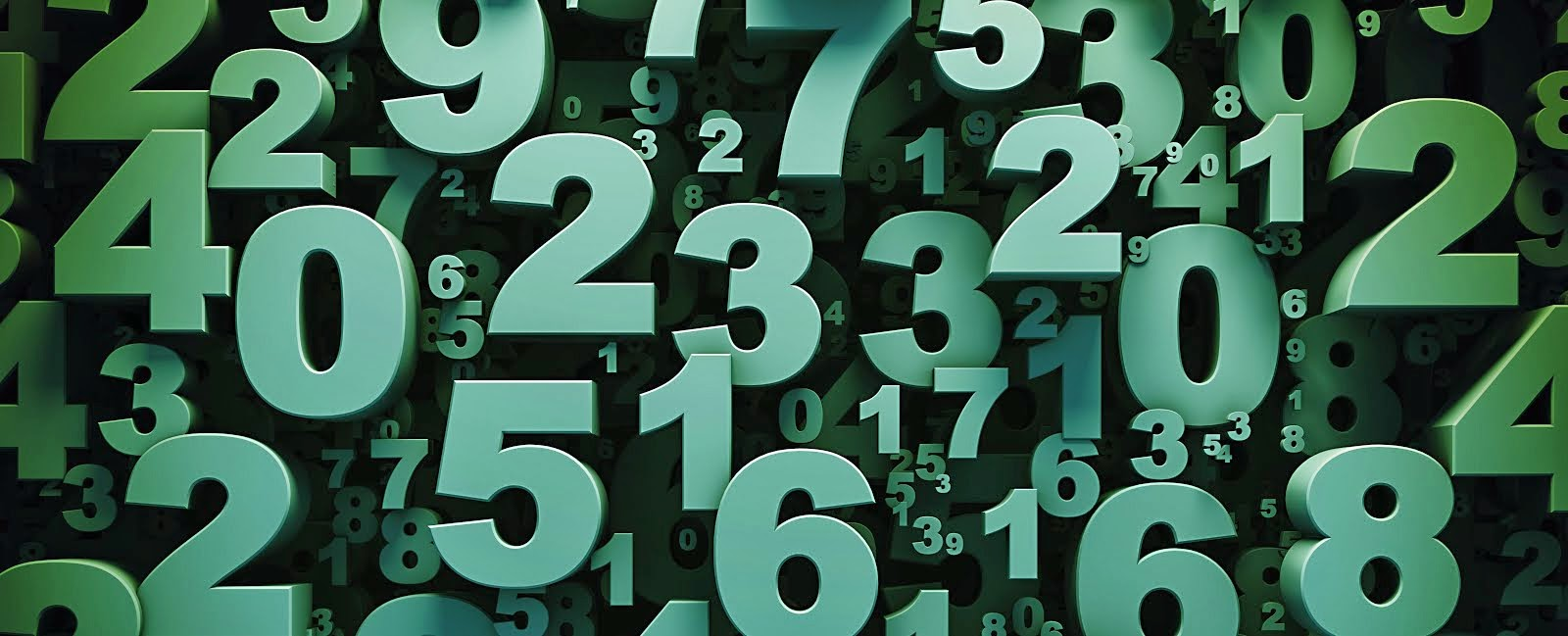.gsc-search-box { } Register for extra features!    LogonTrivia GamesBlogScoreboardsActivityCelebrity TriviaBiographiesPlayers
 Trivia Quiz - Number Quiz - Part 3 More questions where the answers are numbers based on cultural, mathematical and historical knowledge. Quiz Number: 5849 Date Submitted: April 05, 2021 Quiz Categories: Culture Quiz Type: General Quiz Author: grant228 Average Score: 86.7 percent Times Taken: 3 times Taken by Registered Users: 1 Click here for the code snippet to embed this quiz in your website.(Image Source: widehdwallpapers.blogspot.com)

Be sure to register and/or logon before taking quizzes to have your scores saved.

1. I am the number of different phones used in the TV series "Get Smart"; I am the number of the fire station in "Emergency"; Cleopatra became Queen of Egypt in this BC year?
A.   38
B.   44
C.   51
D.   69

2. I am the number of times the Devil tempted Jesus; I am the number of consecutive strikes in bowling that make a "turkey"; I am the fourth Fibonacci number?
A.   2
B.   3
C.   4
D.   5

3. I am the international dialling code for China; I am an American English slang used to indicate that an item is no longer available; I am the code number of Maxwell Smart?
A.   51
B.   67
C.   70
D.   86

4. I am the racing car number of Lightning McQueen in "Cars"; I am the number of times Saturn is heavier than Earth; I am the atomic number of Americium?
A.   40
B.   95
C.   22
D.   74

5. I was the number of days of the original quarantines; I am the minus temperature where Fahrenheit and Celsius are the same; I am the number of squares on a monopoly board?
A.   40
B.   80
C.   60
D.   30

6. I have the most divisors of any whole number under 30; I am the number of points on a backgammon board; I am the number of blackbirds baked into the pie?
A.   16
B.   12
C.   24
D.   18

7. The "Love Bug", Herbie carries this number; I was the year of 20th century Queen Elizabeth II was coronated; "Car 54, Where are You?" was based in this New York Precinct?
A.   50
B.   51
C.   52
D.   53

8. I am the numerical representation if the Yin-yang symbol; I am a song by Deep Purple; I am a semi-prime number i.e. the product of two prime numbers?
A.   35
B.   77
C.   39
D.   69

9. I am the percentage of Earth that is covered by water; I am the sum of three consecutive primes; the BC year when Spartacus was killed?
A.   71
B.   73
C.   77
D.   79

10. I am the number of Earth days in a Mercury year; the speed in m.p.h. the DeLorean in "Back to the Future" must reach; I am the colloquial name for a piano?
A.   52
B.   88
C.   22
D.   72